2006-11-30

# Efectos de la Alta Variabilidad del Tráfico en Trazas de Dispersión de Paquetes de Pueba

## Authors

• Marco Aurelio Alzate Monroy Universidad Distrital Francisco José de Caldas
• Daniel Ortiz Universidad Distrital Francisco José de Caldas
• Daniel Ortiz Universidad Distrital Francisco José de Caldas

## Keywords:

Dependencia de rango amplio, pruebas de pares de paquetes, estimación de tráfico cruzado (es).

4

# Effects of traffic high variability in probing packet dispersion traces

## Marco AlzatePertenece al grupo de investigación GITUD, Universidad DistritalSandra AlmonacidPertenece al grupo de investigación GITUD, Universidad DistritalDaniel OrtizPertenece al grupo de investigación GITUD, Universidad Distrital

### Abstract

In the context of constant-rate fluid-flow traffic estimation, it has been shown that there is a minimum probing traffic rate at which the dispersion exhibits correlation with cross traffic, so both probing packet length and input gap must be adjusted to reach that minimum. However, here we show evidence that, with highly variable traffic, it is possible to have very short probing packets at a very low rate and still get an important correlation between dispersion and traffic, over a long range of measurement time scales, even when the utilization factor is low.

Keywords:
Long range dependence, packet pair probing, cross-traffic estimation

### Resumen

En el contexto de la estimación de tráfico fluido en redes de comunicaciones, se ha demostrado que existe una mínima tasa de tráfico de prueba a la cual las medidas de dispersión están correlacionadas con el tráfico cruzado, de manera que tanto la longitud de los paquetes de prueba como el tiempo de transmisión entre ellos se deben ajustar para alcanzar ese mínimo. En este artículo mostramos evidencia de que, con tráfico variable, es posible usar paquetes de prueba pequeños a una tasa baja conservando una correlación significativa entre las medidas de dispersión y el tráfico, en un amplio rango de escalas, aún con un bajo factor de utilización del enlace.

Palabras clave:
Dependencia de rango amplio, pruebas de pares de paquetes, estimación de tráfico cruzado.

1. Introduction

Several network parameters and traffic conditions can be inferred from packet dispersion measurements . In an active probing scheme, a sender transmits packets of given length at given instants of time and a receiver collects them, taking note of their arrival times. Under heavy load conditions, these measurements can be used to infer the crosstraffic over the tight-link in a path . In other case, although the dispersion measurements will still be lightly correlated with the tight-link cross-traffic, there will not be a formula for an exact inference. So, under these conditions, most techniques prefer to ignore the corresponding measurements.

Pathrate, for example, is an end-to-end capacity measurement tool that sends thousands of probing packet pairs to discover different modes in the bandwidth distribution. Since capacity should not depend on cross-traffic, the hope is that some of those thousands of packets find an idle narrow link, in which case one of the distribution modes will correspond to capacity and not to traffic effects. Pathload. estimates the available bandwidth of an end-to-end path sending probing packets at an increasing rate. When packet dispersions start to increase steadily with rate, we are supposed to be transmitting at the available bandwidth rate. ToPP. and PathChirp. are conceptually similar to pathload, but they try to be more efficient in searching the knee of the rate/delay curve. TOPP separates probing packets in time and estimates available bandwidth through the average of dispersion measures observed at the receiver. Pathchirp uses an exponentially increasing rate chirp probing train so that, with a single train, it probes the network over a range of rates. Both ToPP and PathChirp achieve similar accuracy to pathload but with smaller overhead.

However, while ToPP ignores the delay correlation information contained in packet trains, Pathchirp tries to exploit this fundamental information. Delphi . is similar to Pathrate in using chirp probing trains but, instead of using the self-congestion principle of pathchirp, it estimates a multifractal wavelet model of the bandwidth. Other measuring techniques and tools include Capprobe, Spruce. and IGI/PTR, among many others.

For a specific example consider IGI/PTR where, initially, the time between probe packet transmissions that gives a high correlation between packet dispersion measurements and cross-traffic rate on the tight link is determined. They assume implicitly that the path is carrying a constant-rate fluid cross-traffic, so it varies the probing transmission rate in order to find the turning point at which the probing-packet dispersion begins to be affected by crosstraffic. We use this criterion considering that, for a highly variable traffic, the turning point can move above and below the constant probe transmission rate, due to cross traffic variations. So, for short-term estimations, we can fix a (rather low) probing transmission rate and use every single dispersion measurement as an important source of information about crosstraffic competing for the tight link. We maintain that, instead of ignoring those measurements during which the tight-link becomes idle, the reduced correlation that still exists between those dispersion measurements and the bursty cross-traffic over that link can be exploited successfully. Indeed, recently we reported an efficient, accurate and timely cross-traffic estimator based on this principle .

In section 2 we show that, for a given average cross-traffic arrival rate, the higher the variability the greater the probability that two consecutive probe packets belong to the same occupation period, no matter whether the variability comes from the variance in the number of arrivals and/ or from the correlation structure of the arrival rate. In section 3 we verify experimentally that, for a given average arrival rate, the higher the variability the greater the correlation between cross-traffic arrival rate and packet dispersion measurements, even when consecutive probe packets do not belong to the same occupation period. Then we conclude the paper and propose some future work.

2. Effects of high variability in the probability of an exact estimation

When a pair of probing packets is sent through an unloaded link of capacity C bps, so that the second packet arrives before the first one has finished its transmission, the dispersion between them at the receiver will be D=L/C, where L is the packet length in bits. However, if there is some cross-traffic competing for the link usage, the measured dispersion will be given by D=(L+X)/C, where X is the number of cross-traffic bits received during the transmission of the first probe packet. Accordingly, we can estimate the average cross-traffic rate, in bits per second, λ = (D.C- L) /(L/C).

If the inter-arrival time is greater than L/C, say T, there will be several possibilities. In the easiest case, the queue does not empty between the departure of the first packet and the arrival of the second packet, in which case the dispersion D will be L/C plus the time taken to transmit the cross-traffic that arrived during T. Consequently, we can estimate the average cross-traffic rate during that period of length T as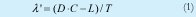Another case is when there is no cross-traffic, in which case both packets find an empty queue and the dispersion is D = T. However, this dispersion measure occurs whenever the crosstraffic is small enough to be completely transmitted between probe packet arrivals (among other unlikely events, as the case in which the queue does not empty and the average arrival rate is exactly C - L/T, or when both probing packets find the same number of bits in queue). In other cases, when one or both packets find a non-empty queue but there are empty periods between the departure of the first packet and the arrival of the second packet, the dispersion will be a random variable more or less correlated with the cross-traffic process, depending on the fraction of time the queue was empty.

So, in order for Equation (1) to be an exact estimator of the cross-traffic arrival rate, the queue should not empty between the departure of the first packet and the arrival of the second packet, i.e. they must belong to the same occupation period of the channel. For this to happen we need one of the following conditions:

1. The first packet finds more than CT-L bits in the queue. In this case, even if there is no cross traffic during T, by the time the first packet is done with its transmission, the second packet will be already in queue.

2. The first packet finds X0 bits in the queue, with 0 d X0 < CT-L, and the cross traffic generates X1 > CT-L- X0 bits during the period (X0+L)/C.

3. The first packet finds X0 bits in the queue, with 0 d X0 < C×T-L, and the cross traffic generates X1 < C×T - L - X0 bits during the period (X0+L)/C and X2 > C×T - L - X0 - X1 bits during the period X1/C.

4. Etc.

Let F1(×) be the stationary CDF of the length of the queue in bits (as seen by the first probe packet) and F2(×;t) be the CDF of the number of arriving bits during t seconds. The above decomposition leads to the following recursive expression for the probability of a pair of probing packet belonging to the same occupation period of the system: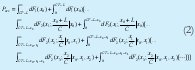Next we will evaluate iteratively the expression above under different conditions of variability, where the variability will be measured both as the variance of an independent traffic process, and as the correlation structure of a Long Range Dependent process. In any case, the evaluation converges after a few iterations.

2.1 Effects of variance

Consider a discrete time channel in which the time unit is the (deterministic) service time of each packet. The number of arrivals during a time unit is an independent random variable with average , which is the utilization factor of the channel. Probing packets of length zero are sent every TN units of time.

We consider two types of independent arrivals. In the first one, the number of arrivals in a time unit obeys a Poisson distribution with mean, characterized by an exponentially decaying tail. The second one is a Pareto-like distribution given by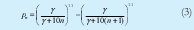which exhibits a power-law decaying tail with finite mean and infinite variance. To obtain equation (3) we consider a continuous Pareto distribution with parameters a= 1.1 and b=y, and consider the probability of unit intervals, as shown in equation (4).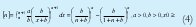Figure 1 shows the probability that two consecutive probing packets belong to the same occupation period as a function of, for both types of arrivals, according to equation (2). The high variability of the traffic, represented here by a heavy tailed distribution, leads to a better cross traffic estimation because equation (1) is exact for a longer period of time compared to an exponentially decreasing tail distribution.

2.2 Effects of long-range-dependence

To consider a high variability represented with a long-range-dependent arrival process we can use, for example, a fractional Brownian motion {A(t), t0} with Hurst parameter 0.5 < H < 1 to model the number of arrivals in the inter val (0, t]. Figure 2 shows the corresponding results for the probability that two consecutive probing packets belong to the same occupation period as a function of , for different Hurst parameters and different probing rates, according to equation (2). The small probabilities (as compared to those of figure 1) are easily understood when we consider both the fluid flow arrivals and the fact that we used very small variances in order to avoid negative samples. This way, these results are due exclusively to the LRD property of the traffic. Clearly, the probability increases with H, the source of variability we wanted to test.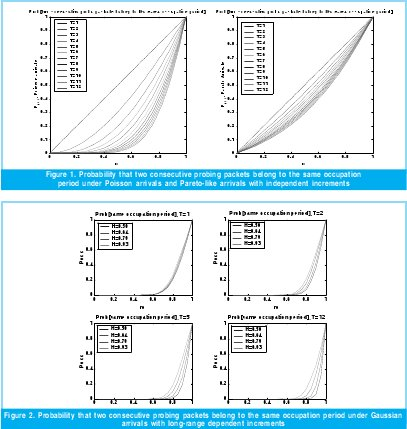3.Effects of variability in the correlation between cross-traffic and dispersion measurements

We have just evaluated equation (2) for traffic with high variance but independent increments, and for a long range dependent traffic but with very small variance. Now we consider the combined effect of variance and LRD through simulation experiments of a single queue, as shown in Figure 3. A C-bps link carries a cross-traffic, characterized by a given coefficient of variation and a given Hurst parameter, along with a probing traffic consisting of L-bits long packets sent every T seconds. We want to know how much correlation is there between the average cross-traffic arrival rate between the nth and the (n+1)st probing packets (the nth measurement period), Xn, and the corresponding packet dispersion measure, Dn. In order to allow both a high variance and a high Hurst parameter, we use synthetic traces from a multi-fractal wavelet model. The results, shown in figure 4, correspond to the coefficient of correlation between Xn and Dn as function of the average utilization of the link, ro, and the time-scale measurement, T.

As we increase the variability through the coefficient of variation (C= 1,2,4), the correlation becomes less dependent on the average utilization factor, ro. Similarly, as we increase the variability through the Hurst parameter (H= 0.5, 0.65, 0.8, 0.95), the correlation becomes less dependent on T as well. For example, the case (C=1, H=0.5), roughly a Poisson traffic,exhibits high correlation only for very small measurement timescale, T, and high utilization factors, ro. On the contrary, the case (C=4, H=0.95) exhibits a high correlation for almost any average utilization factor in a wide range of measurement timescales. Correspondingly, as traffic variability increases, we can test the link over a wide range of time scales obtaining a high correlation between the dispersion measures and the cross-trafic arrival rate, even with a low utilization factor.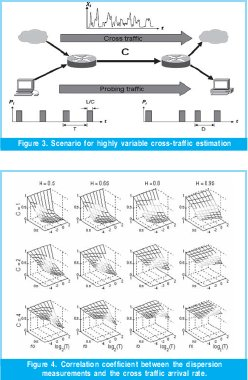4. Conclusions

In estimating highly variable cross-traffic through probing packet pair dispersion measurements, it is possible to use every single measurement as an important source of information, even when both packets belong to different occupation periods. This result brings the possibility of designing high efficiency estimators by avoiding the waste of measurements of current methods. Indeed, in  we report a simple cross-traffic estimator that exploits these characteristics using a computational intelligence approach to infer the cross-traffic rate from the dispersion measurements.

Bibliographic references

 R. Prasad, C. Dovrolis, M. Murray and K.Claffy, «Bandwidth Estimation: Metrics, Measurement Techniques and Tools,» IEEE Network Magazine, Nov./Dec. 2003.

 C.Dovrolis, P.Ramanthan and D.Moore. «Packet Dispersion Techniques and a Capacity Estimation Methodology» 2004.

 N.Hu and P.Steenkiste, «Evaluation and Characterization of Available Bandwidth Probing Techniques» IEEE JSAC, Aug.,2003.

 V.Ribeiro, R.Riedi, R.Baraniuk, J.Navratil and L.Cottrell. «PathChirp: Efficient Available bandwidth Estimation for Network Paths», 2003

 R.Ribeiro, M.Coates, R.Riedi, S. Sarvotham, B.Hendricks, and R.Baraniuk, «MultiFractal Cross-Traffic Estimation», 2000

 M.Melander, M.Bjorkman and P.Gunningberg. «A new End-to-End probing and Analysis Method for Estimating Bandwidth Bottlenecks», 2000

 M.Jain and C.Dovrolis «End-to-End Available Bandwidth Measure Methodology, Dynamics and Relation with TCP throughput», IEEE/ACM Trans.Networking, 2003

 M.Alzate, N.Peña and M.Labrador «Neurofuzzy processing of packet dispersion traces for highly variable crosstraffic estimation», Passive and Active Measurements, PAM 2007, Louvaine-la-neuve, Belgium, 2007.

 R.Kapoor, L.Chen, C.Li, M.Gerla, MSanadidi «CapProbe: A Simple and Accurate Capacity Estimation Technique» SIGCOMM 2004

 J.Strauss, D.Katabi, F.Kaashoek, and B.Prabhakar, «Spruce: A Lightweight End-to-End Tool for Measuring Available Bandwidth»

Marco Alzate